Courses

# Test Level 1: Solid State

## 25 Questions MCQ Test Chemistry Class 12 | Test Level 1: Solid State

Description
This mock test of Test Level 1: Solid State for Class 12 helps you for every Class 12 entrance exam. This contains 25 Multiple Choice Questions for Class 12 Test Level 1: Solid State (mcq) to study with solutions a complete question bank. The solved questions answers in this Test Level 1: Solid State quiz give you a good mix of easy questions and tough questions. Class 12 students definitely take this Test Level 1: Solid State exercise for a better result in the exam. You can find other Test Level 1: Solid State extra questions, long questions & short questions for Class 12 on EduRev as well by searching above.
QUESTION: 1

### Substances that are strongly attracted by applied magnetic field and can be permanently magnetized are

Solution:

Ferromagnetic substance get permanently magnetised in applied Magnetic Field.

QUESTION: 2

### Defects in solids can be studied using

Solution:

All these can be used to detect defects in solid.

QUESTION: 3

### Which type of solid conduct electricity in molten state but not in solid state?

Solution:

In molten state ions can exist so they can conduct electricity

QUESTION: 4

Point defect is also known as

Solution:

Point defect is zero dimensional defect.

QUESTION: 5

Yellow colour of NaCl crystals in sodium vapour is due to

Solution:

This yellow colour is due to metal excess defect of anionic valency.

Na+ion deposited on the surface of crystal of NaCl . The Cl-ion diffused to form NaCl. This happens by loss of electron from Na to Na+.

The released electro diffused into the crystal and occupy anionic site called F center which imparts yellow colour to NaCl.

QUESTION: 6

Dislocation defect is also known as

Solution:

In frenkel defect cation gets dislocated from its position.

QUESTION: 7

Solid X is a very hard electrical insulator in solid as well as in molten state. It melts at extremely high temperature. Solid X is a

Solution:

Covalent solid doesnot conduct electricity in molten and solid state.

QUESTION: 8

Nature of binding forces present in carbondioxide molecules in solid state are?

Solution:

O=C=O<−>−O−C+=O

QUESTION: 9

What type of interaction hold the molecules together in a polar molecular solid?

Solution:

Polar molecule will act as a dipole.

QUESTION: 10

An atom located at the body center of a cubic unit cell is shared by

Solution:

It is not been shared.

QUESTION: 11

Ionization enthalpy is lowest in

Solution:

Metals have low ionization energy because of there tendency to lose electrons.

QUESTION: 12

The coordination number of AB having rock salt geometry is

Solution:

NaCl has rock salt structure. Coordination No. is 6.

QUESTION: 13

MgO has the structure of NaCl. The coordination number of the ions in MgO is

Solution:

Coordination No. in NaCl is 6.

QUESTION: 14

Type of stoichiometric defect shown by ZnS is

Solution:

ZnS shows Frenkel defect.

QUESTION: 15

A metal crystallizes with a face-centered cubic lattice. The edge of the unit cell is 408 pm. The diameter of the metal atom is

Solution: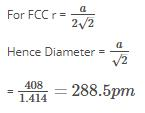QUESTION: 16

An element forms a cubic unit cell with edge length 405 pm. Molar mass of this element is 2.7 X 10-2 Kg/mol and its density is given as 2.7 X 10Kg/m3. How many atoms of this elements are present per unit cell.

Solution: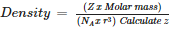QUESTION: 17

A group of 14 element is converted into n – type semiconductor by dopping it with

Solution:

Group 15 doping on group 14 element forms n type semiconductor due to excess electrons.

QUESTION: 18

Group 14 element is converted to p – type semiconductor by dopping it with

Solution:

Doping of group 14 elements with group 13 forms p type semiconductor.

QUESTION: 19

Giant ionic structures is also name given to

Solution:

Explanation:

• An ionic compound is a giant structure of ions.
• The ions have a regular, repeating arrangement called an ionic lattice .
• The lattice is formed because the ions attract each other and form a regular pattern with oppositely charged ions next to each other.
QUESTION: 20

A substance forms face centered cubic crystals. Its density is 1.984 g/cm3 and the length of the edge of the unit cell is 630 pm. Calculate the molar mass in g/mol?

Solution: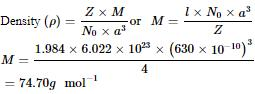QUESTION: 21

The hardest substance among the following is

Solution:

It has network Structure.

QUESTION: 22

The following diagram shows :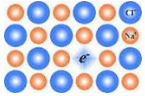Solution:

Extra electron is present at anionic site.

QUESTION: 23

In n – type semiconductor current is carried by

Solution:

N type semiconductors have excess electrons.

QUESTION: 24

The following diagram shows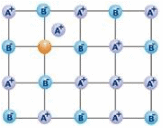Solution:

Cation occupies the interstitial site.

QUESTION: 25

Which transition metal oxide has appearance and conductivity like that of copper?

Solution:

Rhenium oxide and cooper oxide have same appearance and conductivity.